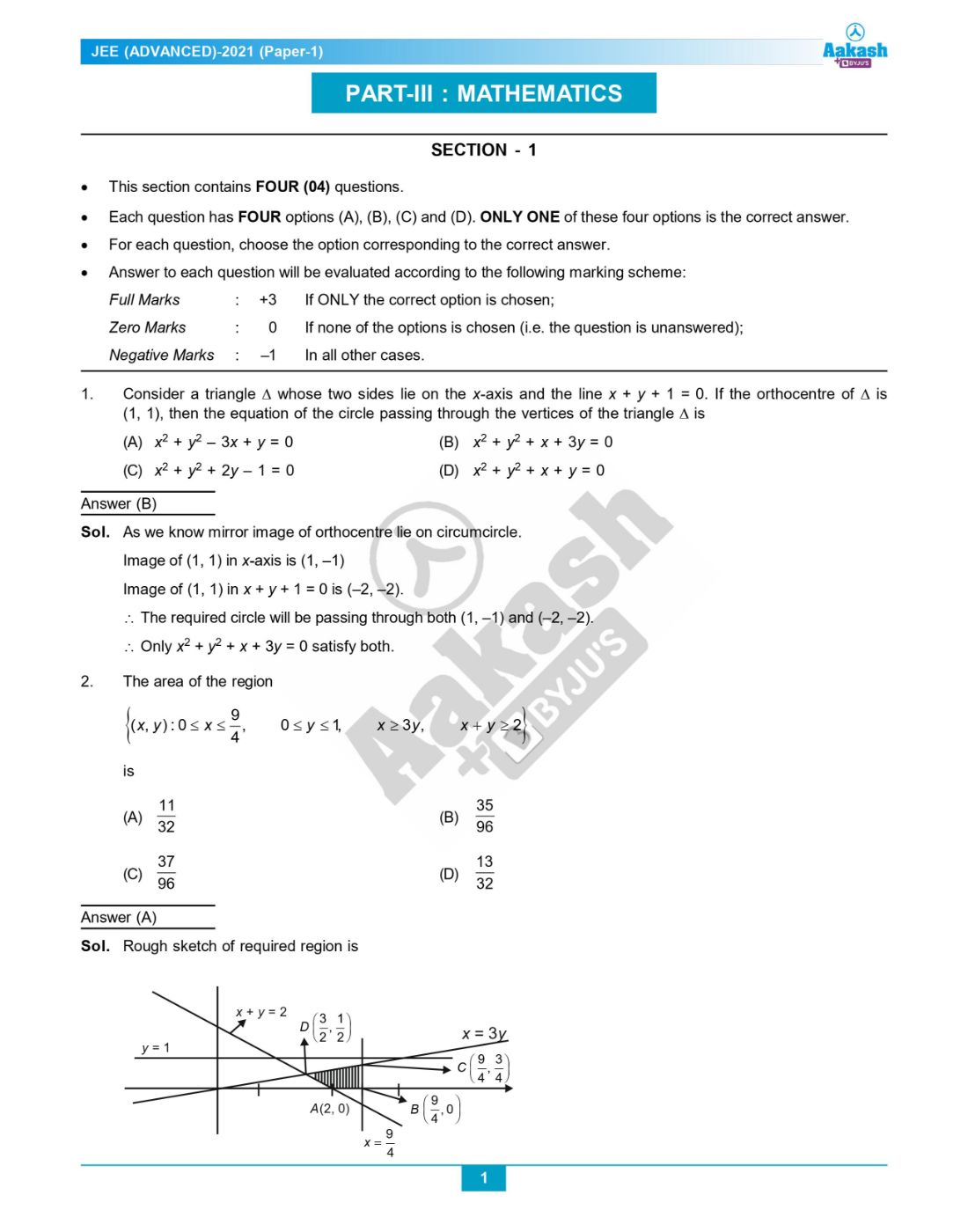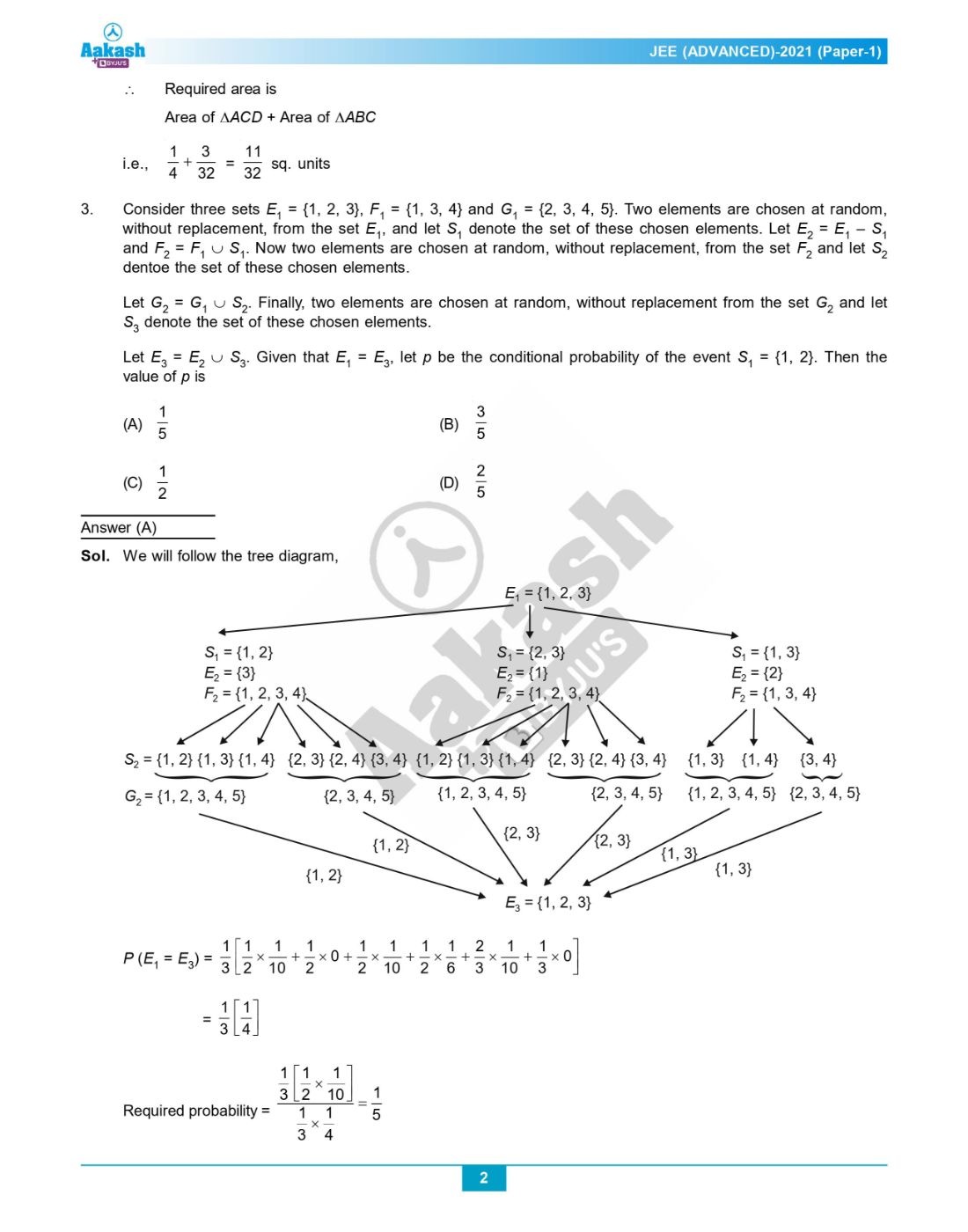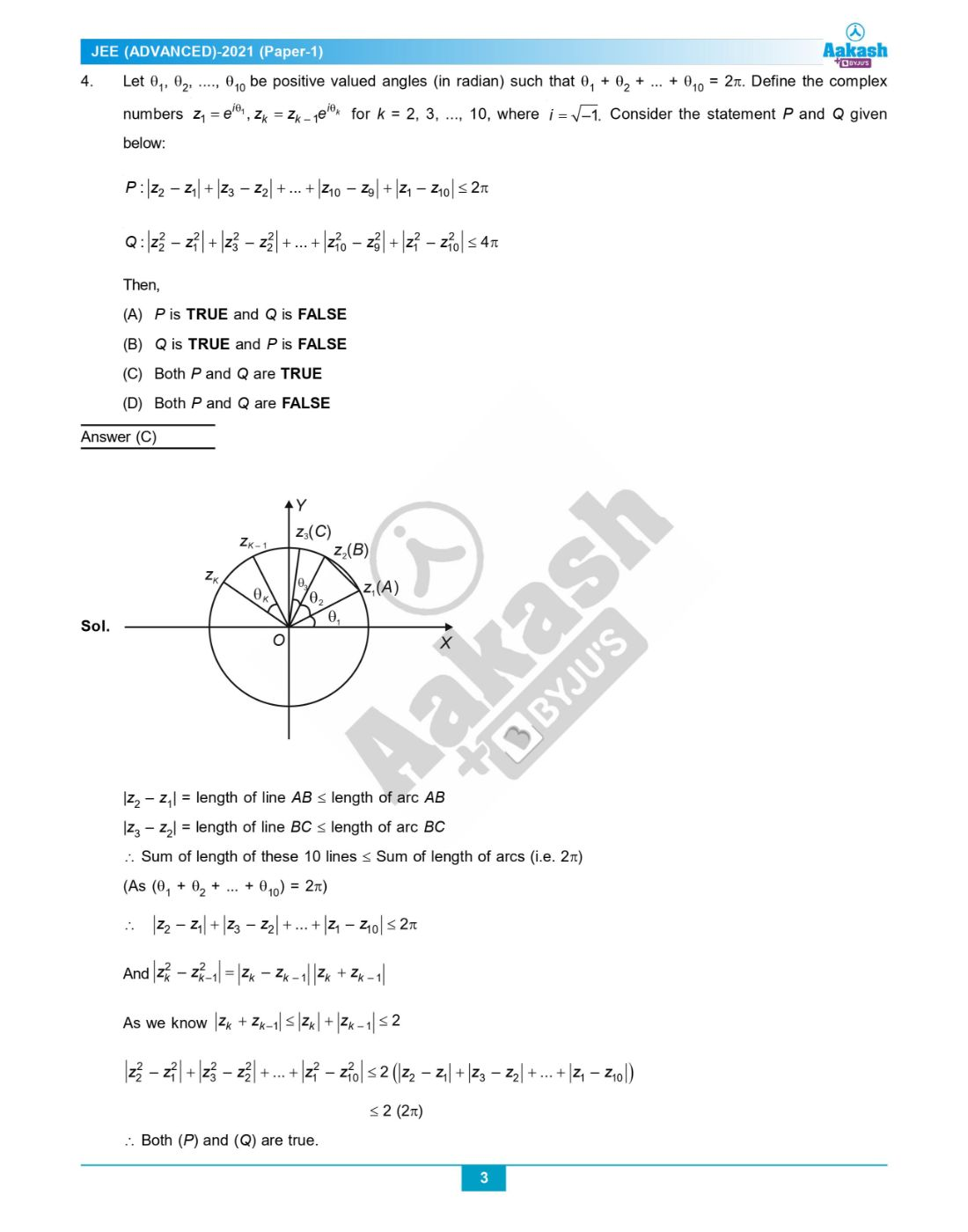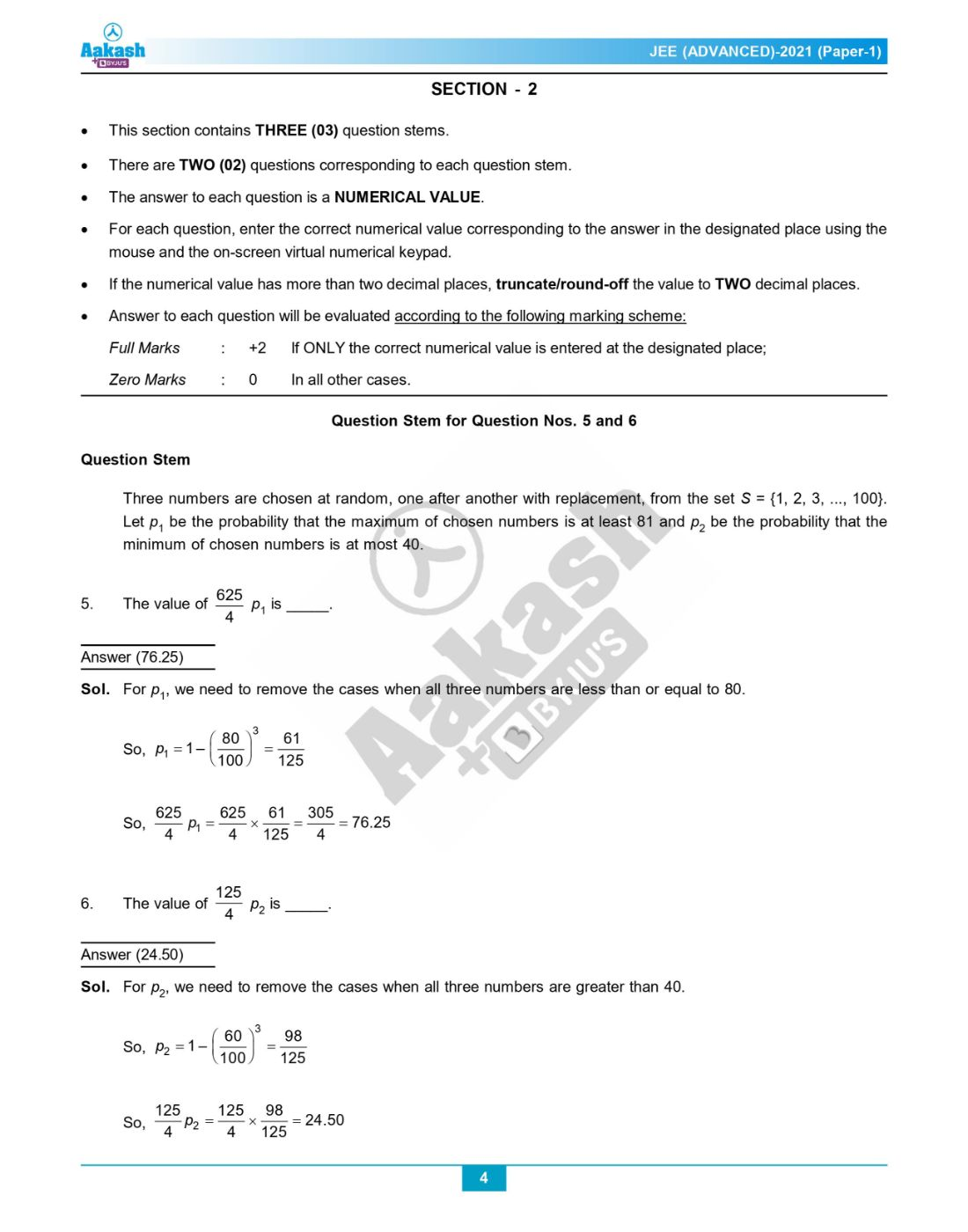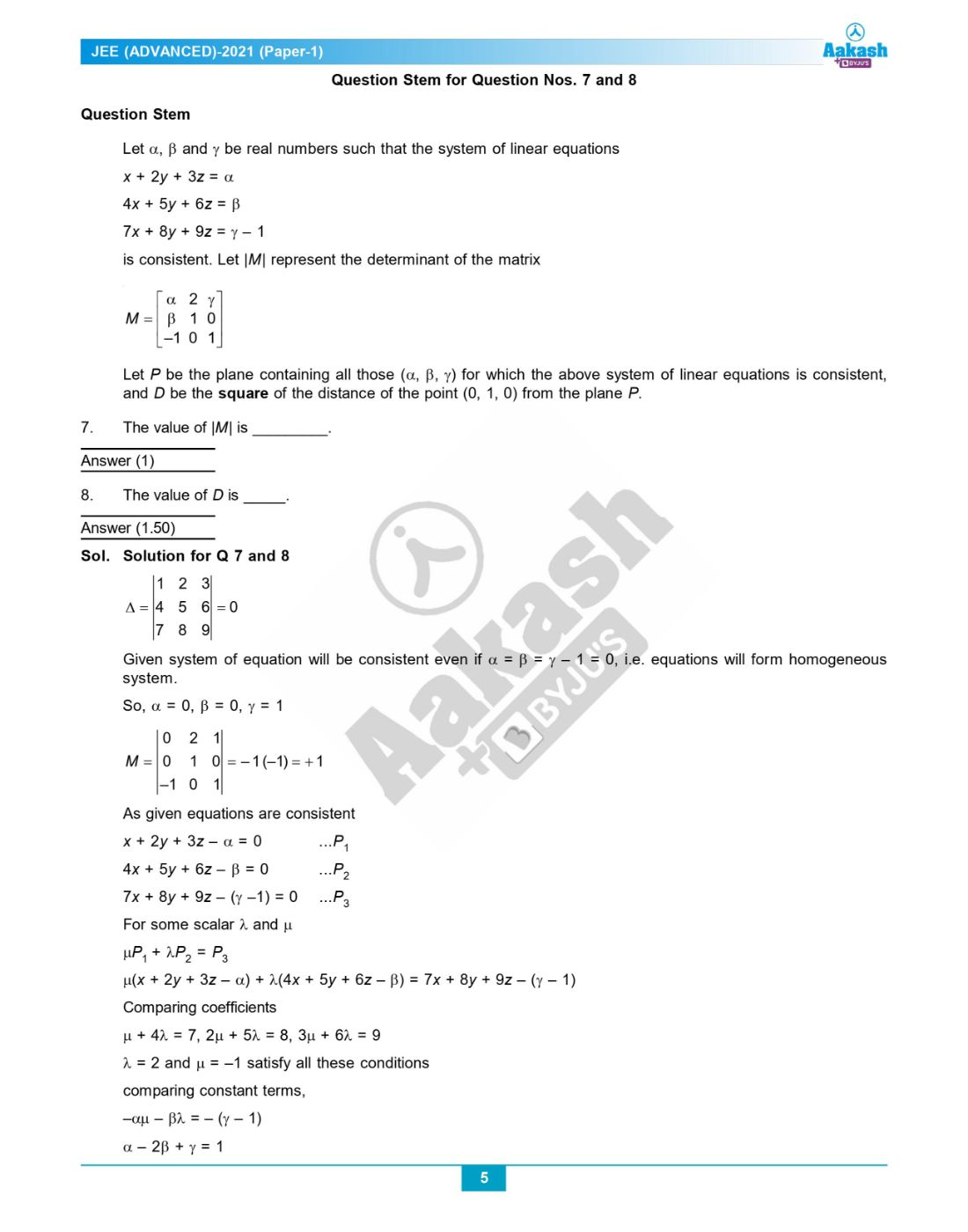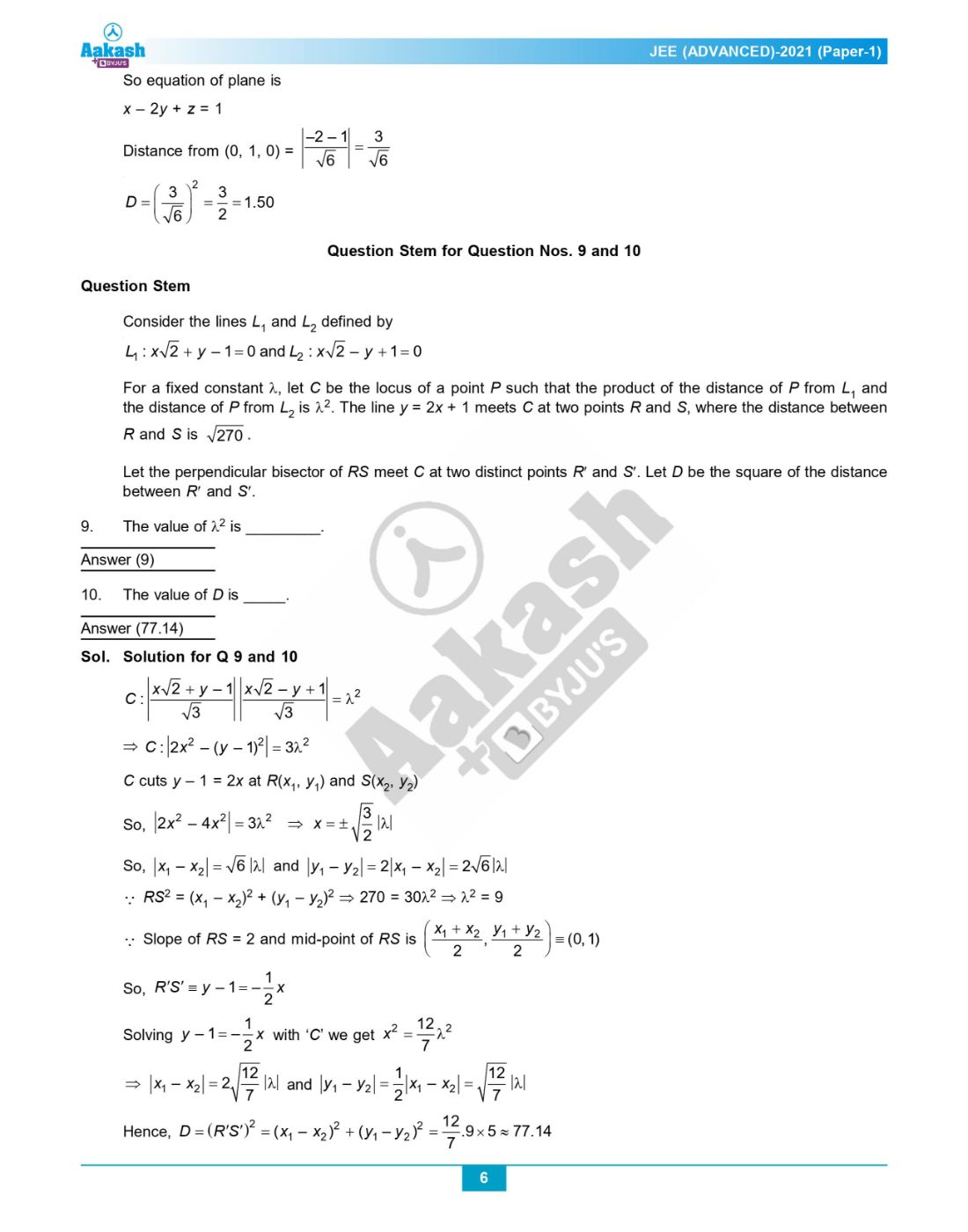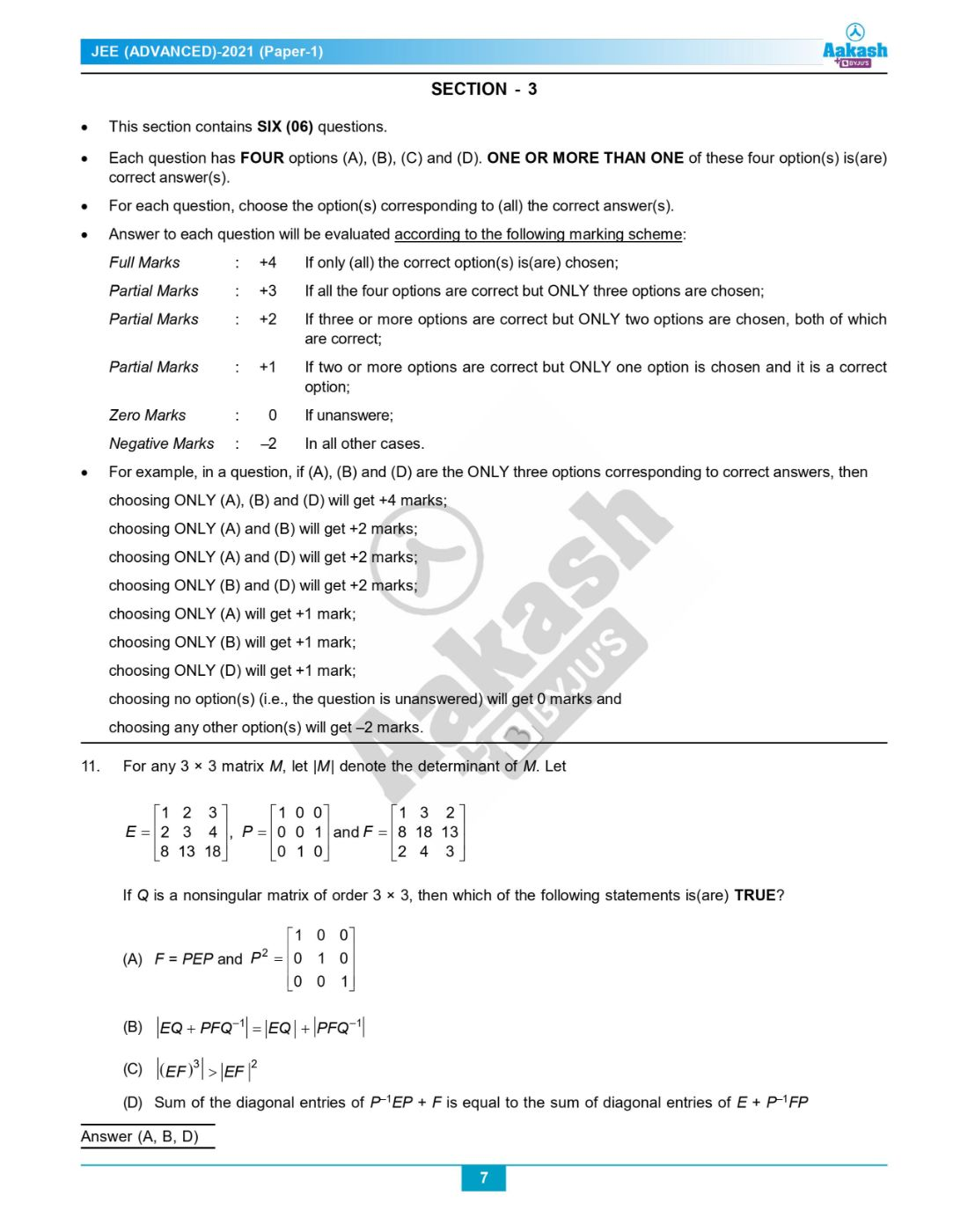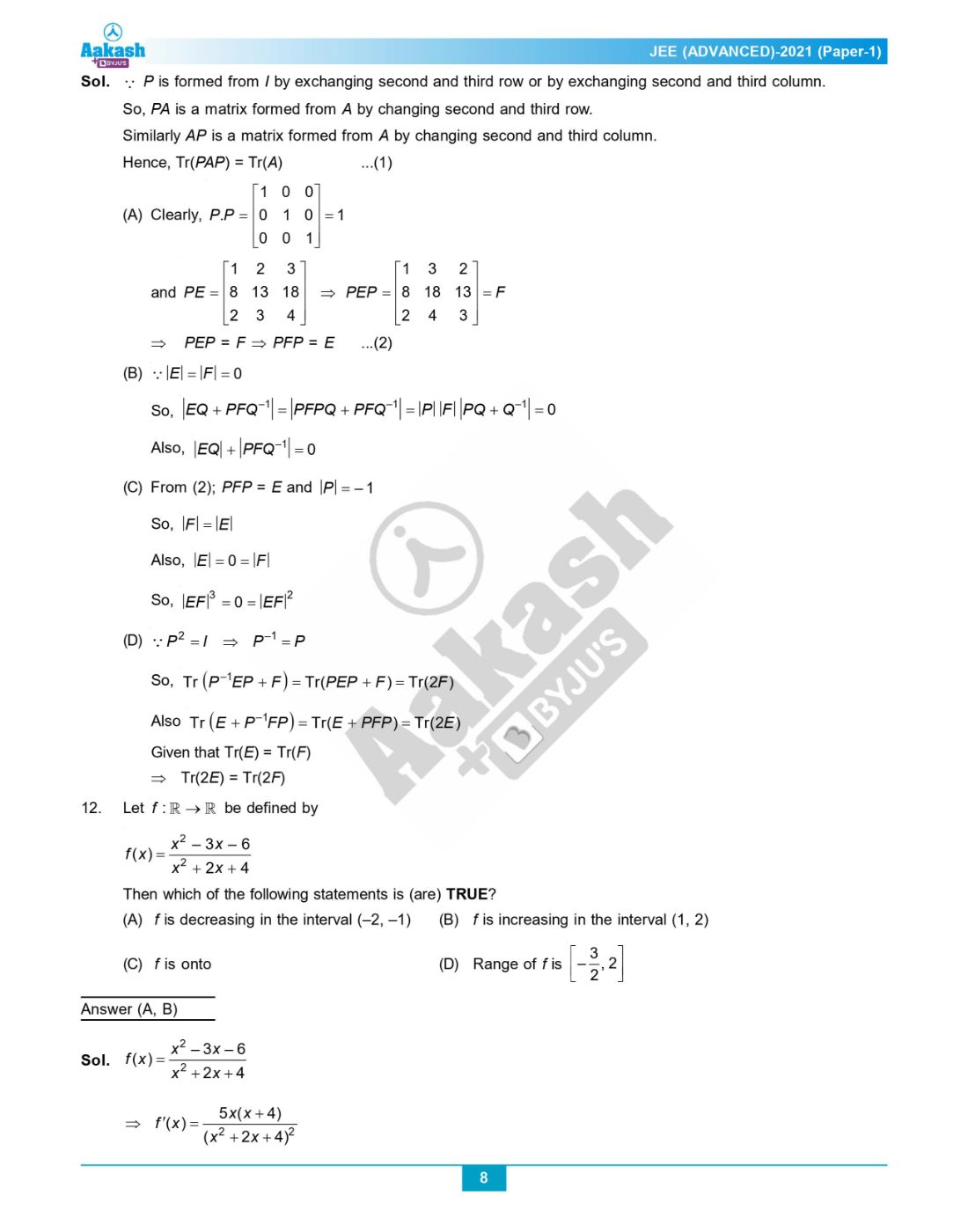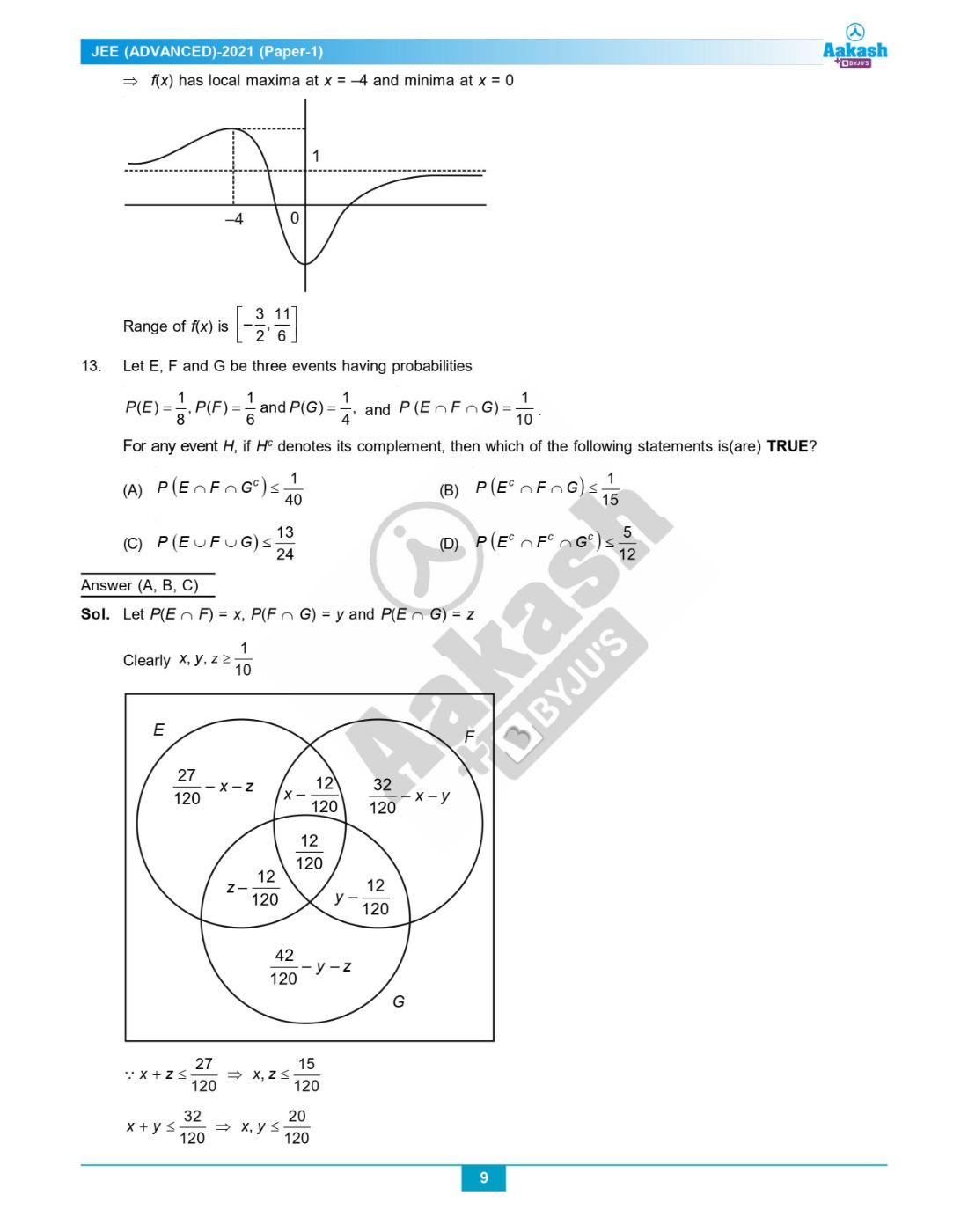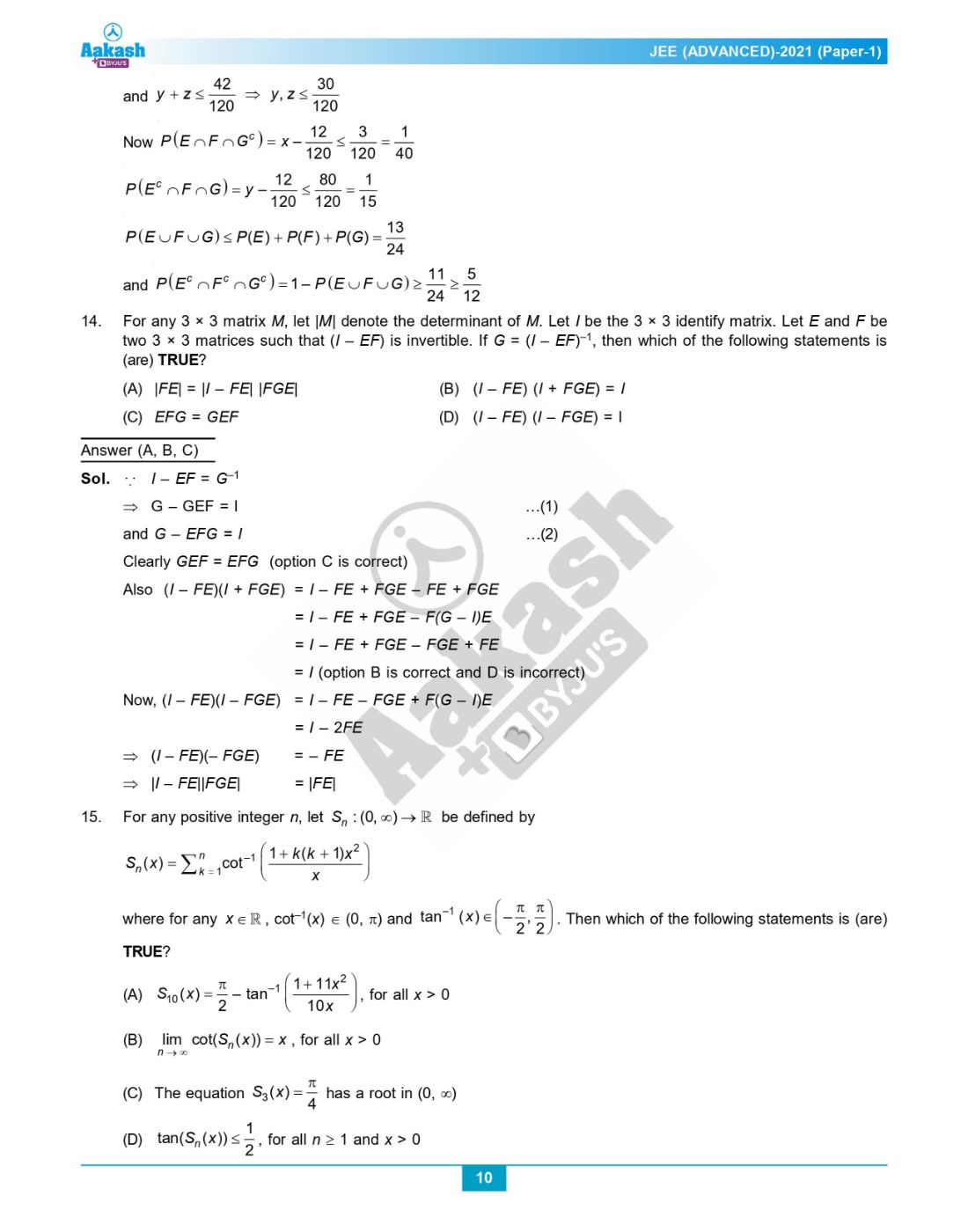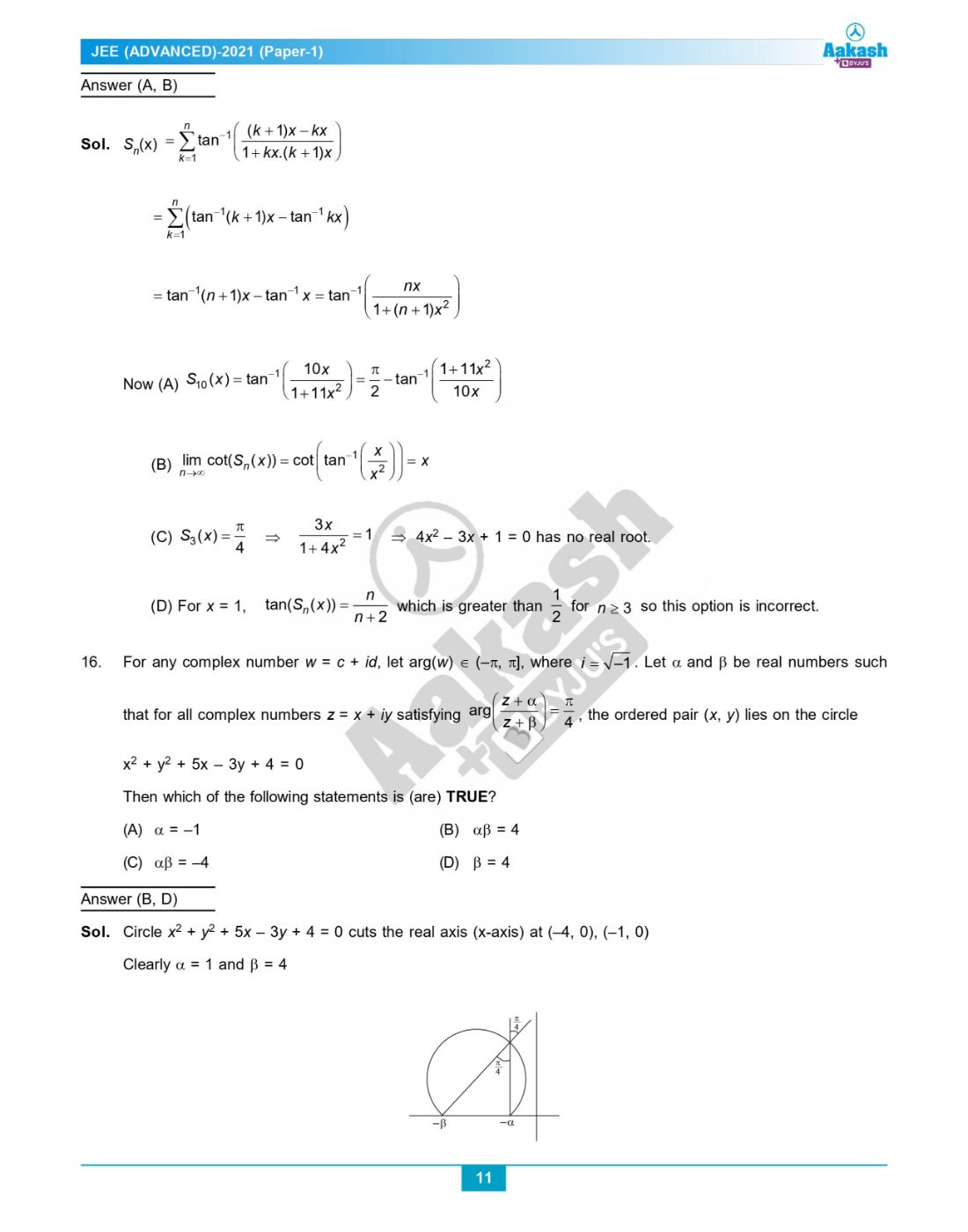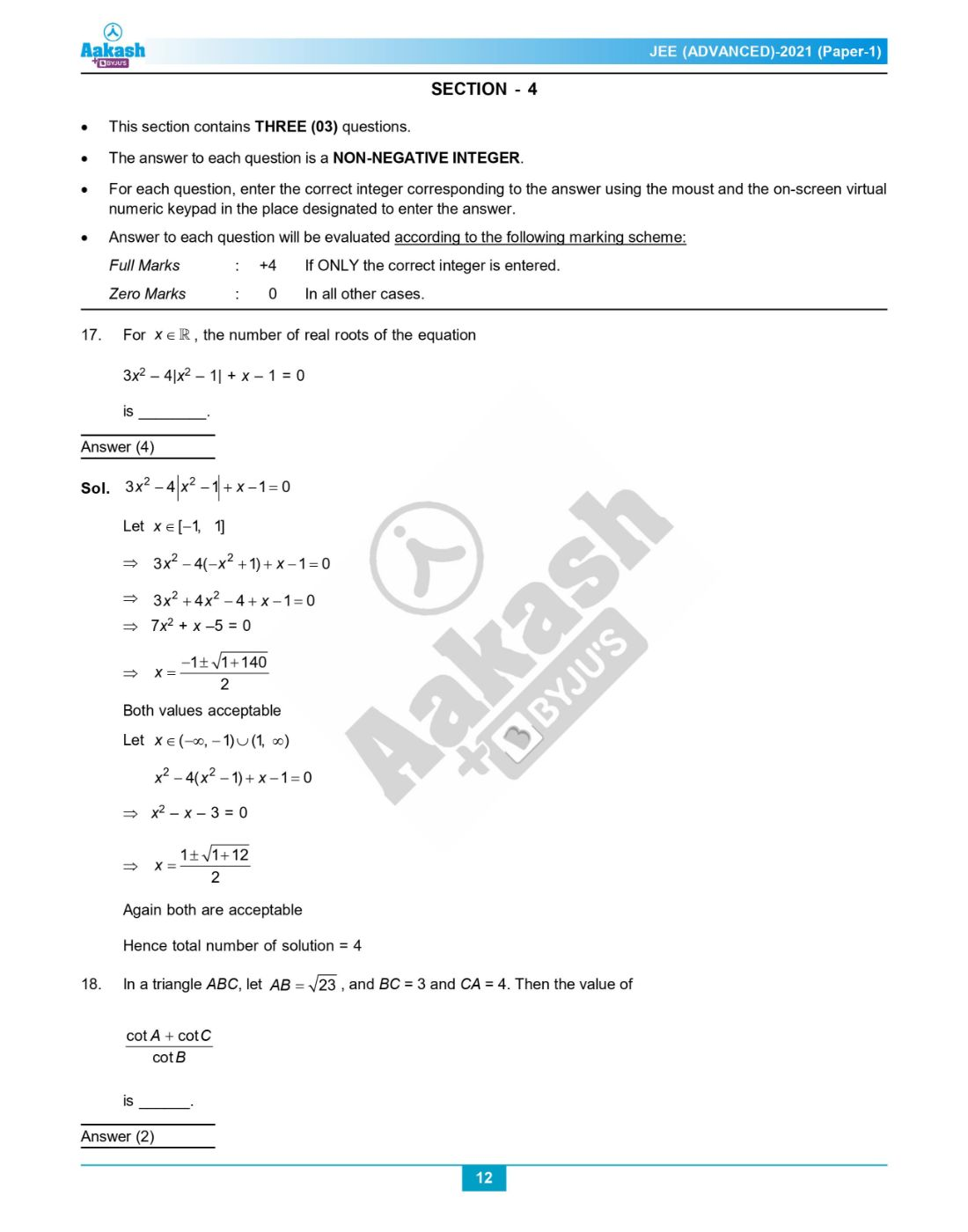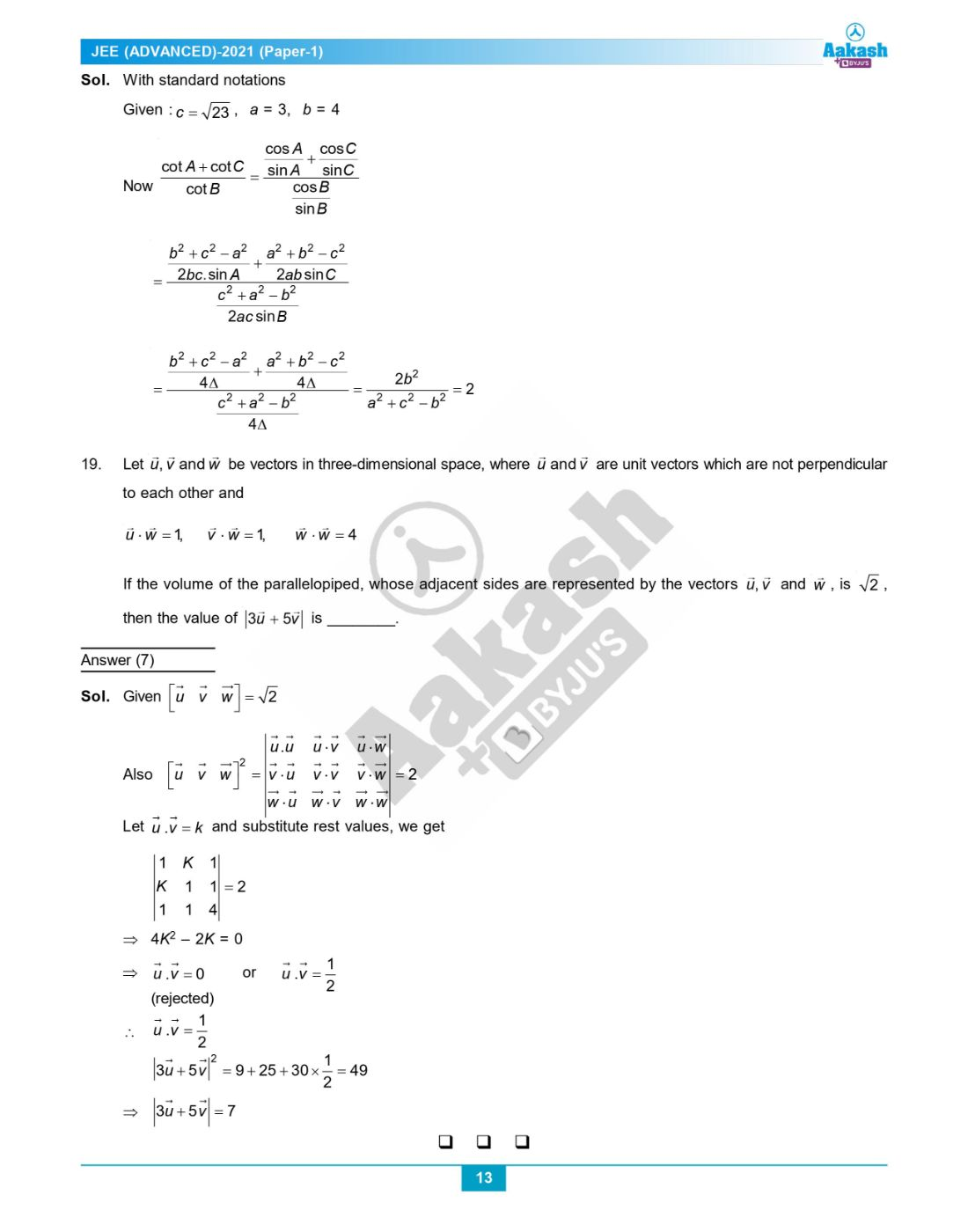Know more about the Repeaters Batch Know more about the Repeaters Batch

# JEE Advanced Question Paper 2021 Maths Paper 1

JEE Advanced Question Paper 2021 Maths Paper 1 is available for reference on this page. The PDF download option is also provided here. Candidates can instantly download the JEE Advanced Question Paper 2021 Maths Paper 1 PDF and use it for offline reference. The solutions are also provided for each question. Going through the paper and the solutions will enable students to learn the right method and logic to answer any type of question in the upcoming exam.

The top 2,45,00 students in JEE Main are qualified to appear for JEE Advanced. The JEE Advanced includes two question papers – Paper 1 and Paper 2. Both the papers together will have a total of 54 questions. These questions are from Chemistry, Physics and Maths. The questions asked in JEE Advanced are based on the Class 11 and Class 12 syllabus.

JEE Advanced 2021 Paper 1 Maths Question Paper

Question 1: Consider a triangle Δ whose two sides lie on the x-axis and the line x + y + 1 = 0. If the orthocenter of Δ is (1, 1), then the equation of the circle passing through the vertices of the triangle Δ is;

a. x2 + y2 – 3x + y = 0
b. x2 + y2 + x + 3y = 0
c. x2 + y2 + 2y – 1 = 0
d. x2 + y2 + x + y = 0

As we know mirror image of the orthocenter lies on the circumcircle.

Image of (1, 1) in x-axis is (1, -1)

Image of (1, 1) in x + y + 1 = 0 is (-2, -2).

∴ The required circle will be passing through both (1, -1) and (-2, -2).

∴ Only x2 + y2 + x + 3y = 0 satisfy both.

Question 2: The area of the region {(x, y): 0 ≤ x ≤ 9/4, 0 ≤ y ≤ 1, x ≥ 3y, x + y ≥ 2}

a. 11/32
b. 35/96
c. 37/96
d. 13/32

A rough sketch of the required region is;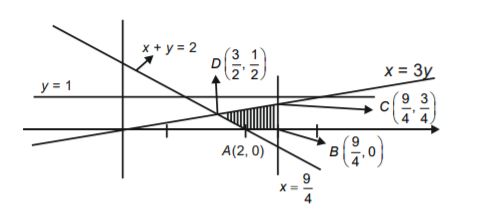∴ Required area is area of ΔACD + Area of ΔABC

i.e (¼) + (3/32) = 11/32 sq.units.

Question 3: Consider three sets E1 = {1, 2, 3}, F1 = {1, 3, 4} and G1 = {2, 3, 4, 5}. Two elements are chosen at random, without replacement, from the set E1, and let S1 denote the set of these chosen elements. Let E2 = E1 – S1 and F2 = F1 ⋃ S1. Now two elements are chosen at random, without replacement, from the set F2 and let S2 dentoe the set of these chosen elements.

Let G2 = G1 ⋃ S2. Finally, two elements are chosen at random, without replacement from the set G2 and let S3 denote the set of these chosen elements. Let E3 = E2 ⋃ S3. Given that E1 = E3, let p be the conditional probability of the event S1 = {1, 2}. Then the value of p is;

a. 1/5
b. 3/5
c. 1/2
d. 2/5

We will follow the tree diagram,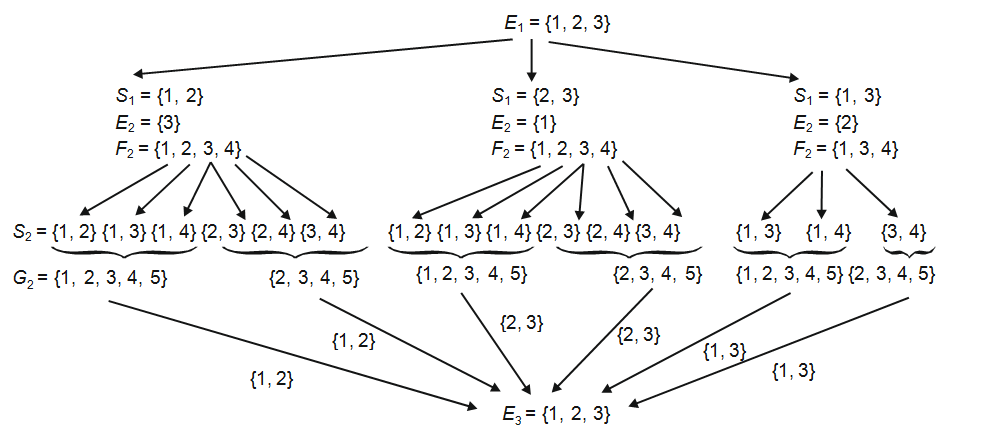P(E1 = E3) = ⅓ [(½ × 1/10) + (½ × 0) + (½ × 1/10) + (½ × ⅙) + (⅔ × 1/10) + (⅓ × 0)]

= ⅓ (¼)

Required probability = ⅓ (½ × 1/10)/(⅓ × ¼ )

= 1/5

Question 4: Let θ1, θ2, …., θ10 be positive valued angles (in radian) such that θ1+ θ2+ ….+ θ10 = 2π. Define the complex numbers z1 =

$$\begin{array}{l}e^{i\theta _{1}}\end{array}$$
, zk =
$$\begin{array}{l}z_{k-1}e^{i\theta _{k}}\end{array}$$
for k = 2, 3, …, 10, where i = √-1. Consider the statement P and Q given below:

P: |z2 – z1| + |z3 – z2| + …. +|z10 – z9| + |z1 – z10| ≤ 2π

Q: |z22 – z12| + |z32 – z22| + …. +|z102 – z92| + |z12 – z102| ≤ 4π

Then,

a. P is TRUE and Q is FALSE
b. Q is TRUE and P is FALSE
c. Both P and Q are TRUE
d. Both P and Q are FALSE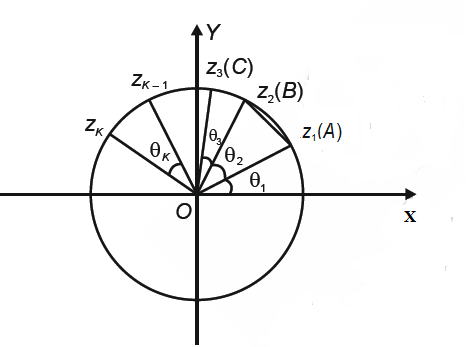|z2 – z1| = length of line AB ≤ length of arc AB

|z3 – z2| = length of line BC ≤ length of arc BC

∴ Sum of length of these 10 lines ≤ Sum of length of arcs (i.e. 2π)

(As θ1+ θ2+ ….+ θ10 = 2π)

∴ |z2 – z1| + |z3 – z2| + …. + |z1 – z10| ≤ 2π

And |zk2 – z2k-1| = |zk – zk-1| |zk + zk-1|

As we know |zk + zk-1| ≤ |zk| + |zk-1| ≤ 2

|z22 – z12| + |z32 – z22| + …. + |z12 – z102| ≤ 2(|z2 – z1| + |z3 – z2| + …. + |z1 – z10|) ≤ 2(2π)

∴ Both P and Q are true.

Question Stem for Question Nos. 5 and 6

Three numbers are chosen at random, one after another with replacement, from the set S = {1, 2, 3, …, 100}. Let p1 be the probability that the maximum of chosen numbers is at least 81 and p2 be the probability that the minimum of chosen numbers is at most 40.

Question 5: The value of (625/4)p1 is

For p1, we need to remove the cases when all three numbers are less than or equal to 80.

So p1 = 1 – (80/100)3

= 61/125

So (625/4)p1 = (625/4)× 61/125

= 305/4

= 76.25

Question 6: The value of (125/4)p2 is

For p2, we need to remove the cases when all three numbers are greater than 40.

So p2 = 1 – (60/100)3

= 98/125

So (125/4)p2 = (125/4)×98/125

= 24.50

Question Stem for Question Nos. 7 and 8

Let α, β and γ be real numbers such that the system of linear equations

x + 2y + 3z = α

4x + 5y + 6z = β

7x + 8y + 9z = γ – 1 is consistent.

Let |M| represent the determinant of the matrix.

M =

$$\begin{array}{l}\begin{bmatrix} \alpha & 2 &\gamma \\ \beta & 1&0 \\ -1 & 0& 1 \end{bmatrix}\end{array}$$

Let P be the plane containing all those (α, β, γ) for which the above system of linear equations is consistent, and D be the square of the distance of the point (0, 1, 0) from the plane P.

Question 7: The value of |M| is

Question 8: The value of D is

Solution for Q.7 and Q.8

=

$$\begin{array}{l}\begin{vmatrix} 1 & 2 & 3\\ 4&5 &6 \\ 7& 8 & 9 \end{vmatrix}= 0\end{array}$$

Given system of equation will be consistent even if α = β = γ-1 = 0, i.e. equations will form homogeneous system.

So α = 0, β = 0, γ = 1

M =

$$\begin{array}{l}\begin{vmatrix} 0 & 2 & 1\\ 0&1 &0 \\ -1& 0 & 1 \end{vmatrix}\end{array}$$

= -1×(-1)

= 1

As given equations are consistent

x + 2y + 3z – α = 0 …P1

4x + 5y + 6z – β = 0 …P2

7x + 8y + 9z – (γ – 1) = 0 …P3

For some scalar λ and μ

μP1 + λP2 = P3

μ(x + 2y + 3z – α) + λ(4x + 5y + 6z – β) = 7x + 8y + 9z – (γ – 1)

comparing coefficients

μ+ 4λ = 7, 2μ + 5λ = 8, 3μ + 6λ = 9

λ = 2 and μ = -1 satisfy all these conditions

comparing constant terms,

αμ – βλ = – (γ – 1)

α – 2β + γ = 1

So equation of plane is

x – 2y + z = 1

distance from (0, 1, 0) = |(-2-1)/√6| = 3/√6

D = (3/√6)2 = 9/6 = 3/2 = 1.50

Question Stem for Question Nos. 9 and 10

Consider the lines L1 and L2 defined by

L1 : x√2 + y – 1 = 0 and L2 : x√2 – y + 1 = 0

For a fixed constant λ, let C be the locus of a point P such that the product of the distance of P from L1 and the distance of P from L2 is λ2. The line y = 2x + 1 meets C at two points R and S, where the distance between R and S is √270.

Let the perpendicular bisector of RS meet C at two distinct points R’ and S’. Let D be the square of the distance between R’ and S’.

Question 9: The value of λ2 is

Question 10: The value of D is

Solution for Q.9 and Q.10

C: |(x√2 + y – 1)/√3| |(x√2 – y + 1)/√3| = λ2

⇒ C: |2x2 – (y-1)2| = 3λ2

C cuts y-1 = 2x at R(x1, y1) and S(x2, y2)

So |2x2 – 4x2| = 3λ2

⇒ x = ±√(3/2) |λ|

So |x1 – x2| = √6|λ| and |y1 – y2| = 2| x1 – x2| = 2√6|λ|

Since RS2 = (x1 – x2)2 + (y1-y2)2

⇒ 270 = 30λ2

⇒ λ2 = 270/30 = 9

Since slope of RS = 2 and midpoint of RS is ((x1+x2)/2, (y1+y2)/2) ≡ (0, 1)

So R’S’ ≡ y-1 = -½ x

Solving y-1 = -½ x with ‘C’ we get x2 = (12/7) λ2

⇒ |x1-x2| = 2√(12/7) |λ| and |y1-y2| = ½ |x1-x2| = √(12/7) |λ|

Hence D = (R’S’)2 = (x1-x2)2 + (y1-y2)2 = (12/7)9×5 ≈ 77.14

Question 11: For any 3 × 3 matrix M, let |M| denote the determinant of M. Let E =

$$\begin{array}{l}\begin{bmatrix} 1 & 2 & 3\\ 2& 3 &4 \\ 8 & 13 & 18 \end{bmatrix}\end{array}$$
,

P =

$$\begin{array}{l}\begin{bmatrix} 1 & 0 & 0\\ 0& 0 &1 \\ 0 & 1 & 0 \end{bmatrix}\end{array}$$
,

And F =

$$\begin{array}{l}\begin{bmatrix} 1 & 3 & 2\\ 8& 18 &13 \\ 2 & 4 & 3 \end{bmatrix}\end{array}$$

If Q is a nonsingular matrix of order 3 × 3, then which of the following statements is(are) TRUE?

a. F = PEP and P2 =

$$\begin{array}{l}\begin{bmatrix} 1 &0 &0 \\ 0 &1 & 0\\ 0& 0 & 1 \end{bmatrix}\end{array}$$

b. |EQ + PFQ-1 | = |EQ| +|PFQ-1|
c. |(EF)3| > |EF|2
d. Sum of the diagonal entries of P-1EP + F is equal to the sum of diagonal entries of E + P-1FP

P is formed from I by exchanging second and third rows or by exchanging second and third columns.

So, PA is a matrix formed from A by changing the second and third rows.

Similarly, AP is a matrix formed from A by changing the second and third columns.

Hence, Tr(PAP) = Tr(A) …(1)

(a) Clearly P.P =

$$\begin{array}{l}\begin{bmatrix} 1 &0 &0 \\ 0 &1 & 0\\ 0& 0 & 1 \end{bmatrix}=1\end{array}$$

And PE =

$$\begin{array}{l}\begin{bmatrix} 1 & 2&3 \\ 8& 13 & 18\\ 2& 3 & 4 \end{bmatrix}\end{array}$$

⇒ PEP =

$$\begin{array}{l}\begin{bmatrix} 1 & 3&2 \\ 8& 18 & 13\\ 2& 4 & 3 \end{bmatrix}=F\end{array}$$

⇒ PEP = F ⇒ PFP = E …(2)

(b) Since |E| = |F| = 0

So, |EQ + PFQ–1 | = |PFPQ + PFQ–1| = | P| | F| | PQ + Q–1 | = 0

Also, |EQ|+|PFQ–1 | = 0

(c) From (2); PFP = E and |P| = -1

So, |F| = |E|

Also, |E| = 0 = |F|

So, |EF|3 = 0 = |EF|2

(d) since P2 = I ⇒ P –1 = P

So, Tr (P-1EP + F) = Tr(PEP + F) = Tr(2F )

Also Tr (E + P –1FP) = Tr(E + PFP) = Tr(2E )

Given that Tr(E) = Tr(F)

⇒ Tr(2E) = Tr(2F)

Question 12: Let F: R→ R be defined by f(x) = (x2-3x-6)/(x2+2x+4). Then which of the following statements is (are) TRUE?

a. f is decreasing in the interval (-2, -1)
b. f is increasing in the interval (1, 2)
c. f is onto
d. Range of f is [-3/2, 2]

f(x) = (x2-3x-6)/(x2+2x+4)

⇒ f’(x) = 5x(x+4)/(x2+2x+4)2

⇒ f(x) has local maxima at x = -4 and minima at x = 0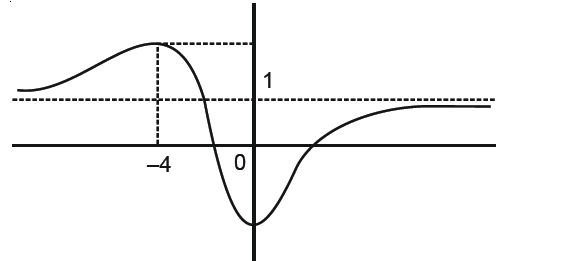Range of f(x) is [-3/2, 11/6]

Question 13: Let E, F and G be three events having probabilities P(E) = 1/8, P(F) = ⅙ and P(G) = ¼, and P(E⋂F⋂G) = 1/10. For any event H, if Hc denotes its complement, then which of the following statements is(are) TRUE?

a. P(E⋂F⋂Gc) ≤ 1/40
b. P(Ec⋂F⋂G) ≤ 1/15
c. P(E⋃F⋃G) ≤ 13/24
d. P(Ec⋂Fc⋂Gc) ≤ 5/12

Let P(E⋂F) = x, P(F⋂G) = y and P(E⋂G) = z

Clearly x, y, z ≥ 1/10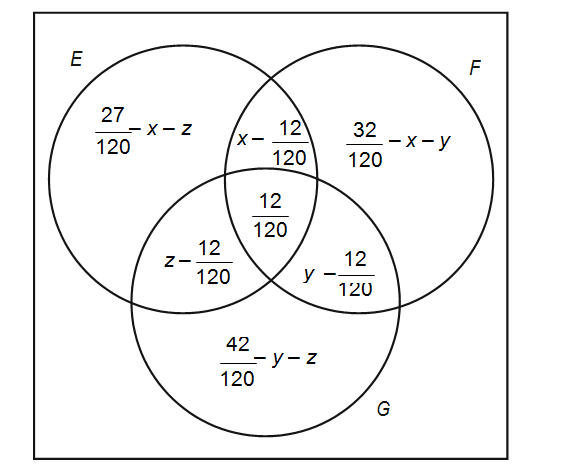Since x + z ≤ 27/120

⇒ x, z ≤ 15/120

x+y ≤ 32/120

⇒ x, y ≤ 20/120

And y + z ≤ 42/120 ⇒ y, z ≤ 30/120

Now P(E⋂F⋂Gc) = x – 12/120 ≤ 3/120 = 1/40

P(Ec⋂F⋂G) = y – 12/120 ≤ 80/120 = 1/15

P(E⋃F⋃G) ≤ P(E) + P(F) + P(G) = 13/24

And P(Ec⋂Fc⋂Gc) = 1 – P(E⋃F⋃G) ≥ 11/24 ≥ 5/12

Question 14: For any 3 × 3 matrix M, let |M| denote the determinant of M. Let I be the 3 × 3 identify matrix. Let E and F be two 3 × 3 matrices such that (I – EF) is invertible. If G = (I – EF)–1, then which of the following statements is(are) TRUE?

a. |FE| = |I – FE| |FGE|
b. (I – FE) (I + FGE) = I
c. EFG = GEF
d. (I – FE) (I – FGE) = I

I – EF = G–1

G – GEF = I …(1)

And G – EFG = I …(2)

Clearly GEF = EFG (option C is correct)

Also (I – FE)(I + FGE) = I – FE + FGE – FE + FGE

= I – FE + FGE – F(G – I)E

= I – FE + FGE – FGE + FE

= I (option B is correct and D is incorrect)

Now, (I – FE)(I – FGE) = I – FE – FGE + F(G – I)E

= I – 2FE

(I – FE)(- FGE) = – FE

|I – FE||FGE| = |FE|

Question 15: For any positive integer n, let Sn: (0, ∞) → R be defined by Sn(x) = ∑k=1n cot-1((1 + k(k+1)x2)/x) where for any x ∈R , cot–1(x) ∈ (0, π) and tan–1( x)∈ (-π/2, π/2). Then which of the following statements is (are) TRUE?

a. S10(x) = π/2 – tan-1(1 + 11x2)/10x, for all x >0
b. lim n→∞ cot(Sn(x)) = x, for all x >0
c. The equation S3(x) = π/4 has a root in (0, ∞)
d. tan(Sn(x)) ≤1/2, for all n≥1 and x > 0

Sn(x) =

$$\begin{array}{l}\sum_{k=1}^{n}\tan^{-1}(\frac{(k+1)x-kx}{1+kx.(k+1)x})\end{array}$$

=

$$\begin{array}{l}\sum_{k=1}^{n}(\tan^{-1}{(k+1)x-\tan^{-1}kx})\end{array}$$

= tan-1(n+1)x – tan-1x

= tan-1(nx/(1+(n+1)x2))

Now,

(a) S10(x) = tan-1(10x/(1+11x2)) = π/2 – tan-1((1+11x2)/10x)

(b) limn→ ∞cot (Sn(x)) = cot (tan-1x/x2) = x

(c) S3(x) = π/4 => 3x/(1+4x2) = 1

⇒ 4x2-3x+1=0 has no real root.

(d) for x = 1, tan(Sn(x)) = n/(n+2) which is greater than 1/2 for n≥3 so this option is incorrect.

Question 16: For any complex number w = c + id, let arg(w) ∈ (-π, π], where i = √-1 . Let α and β be real numbers such that for all complex numbers z = x + iy satisfying arg(z+α)/(z+β) = π/4, the ordered pair (x, y) lies on the circle x2 + y2 + 5x – 3y + 4 = 0. Then which of the following statements is (are) TRUE?

a. α = -1
b. αβ = 4
c. αβ = -4
d. β = 4

Circle x2 + y2 + 5x – 3y + 4 = 0 cuts the real axis (x-axis) at (-4, 0), (-1, 0)

Clearly α = 1 and β = 4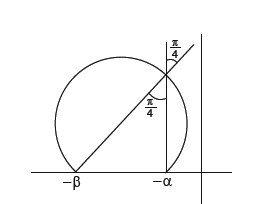Question 17: For x ∈ R, the number of real roots of the equation 3x2 – 4|x2 – 1| + x – 1 = 0 is

3x2 – 4|x2 – 1| + x – 1 = 0

Let x ∈ [-1, 1]

⇒ 3x2 – 4(-x2+1) + x – 1 = 0

⇒ 3x2 + 4x2 – 4 + x – 1 = 0

⇒ 7x2 + x – 5 = 0

⇒ x = -1±√(1+140)/2

Both values are acceptable.

Let x ∈ (-∞, -1) ⋃ (1, ∞)

x2-4(x2-1)+x-1 = 0

⇒ x2-x-3 = 0

⇒ x = 1±√(1+12)/2

Again both are acceptable.

Hence, total number of solution = 4.

Question 18: In a triangle ABC, let AB = √23, and BC = 3 and CA = 4. Then the value of (cot A + cot C)/cot B is;

With standard notations

Given c = √23, a = 3 and b = 4

Now (cot A + cot C)/cot B = (cos A/sin A + cos C/sin C)/(cos B/sin B)

= ((b2+c2-a2)/2bc sin A + (a2+b2-c2)/2ab sin C)/((c2+a2-b2)/2ac sin B)

= ((b2+c2-a2)/4∆ + (a2+b2-c2)/4∆)/((c2+a2-b2)/4∆)

= 2b2/(a2+c2-b2)

= 2

Question 19: Let

$$\begin{array}{l}\vec{u}, \vec{v}\: and \: \vec{w}\end{array}$$
be vectors in three-dimensional space, where
$$\begin{array}{l}\vec{u}\: and \: \vec{v}\end{array}$$
are unit vectors which are not perpendicular to each other and
$$\begin{array}{l}\vec{u} . \vec{w}= 1\end{array}$$
,
$$\begin{array}{l}\vec{v} . \vec{w}= 1\end{array}$$
and
$$\begin{array}{l}\vec{w} . \vec{w}= 4\end{array}$$
. If the volume of the parallelepiped, whose adjacent sides are represented by the vectors u, v and w is √2 , then the value of
$$\begin{array}{l}\left | 3\vec{u} +5\vec{v}\right |\end{array}$$
is;

Given

$$\begin{array}{l}[\vec{u}\: \: \vec{v}\: \: \vec{w}]= \sqrt{2}\end{array}$$

a.so,

$$\begin{array}{l}[\vec{u}\: \: \vec{v}\: \: \vec{w}]^{2}= \begin{vmatrix} \vec{u}.\vec{u} & \vec{u}.\vec{v} &\vec{u}.\vec{w} \\ \vec{v}.\vec{u}& \vec{v}.\vec{v} & \vec{v}.\vec{w}\\ \vec{w}.\vec{u} & \vec{w}.\vec{v} & \vec{w}.\vec{w} \end{vmatrix}=2\end{array}$$

Let

$$\begin{array}{l}\vec{u}.\vec{v}=k\end{array}$$
and substitute rest values, we get

$$\begin{array}{l}\begin{vmatrix} 1 & K & 1\\ K & 1 &1 \\ 1& 1 & 4 \end{vmatrix}=2\end{array}$$

4K2 – 2K = 0

$$\begin{array}{l}\vec{u}.\vec{v }=0\end{array}$$
(rejected)

Or

$$\begin{array}{l}\vec{u}.\vec{v }=\frac{1}{2}\end{array}$$

Therefore

$$\begin{array}{l}\vec{u}.\vec{v }=\frac{1}{2}\end{array}$$
$$\begin{array}{l}\left | 3\vec{u} +5\vec{v}\right |^{2}\end{array}$$
= 9 + 25 + 30(½)

= 49

$$\begin{array}{l}\left | 3\vec{u} +5\vec{v}\right |\end{array}$$
= 7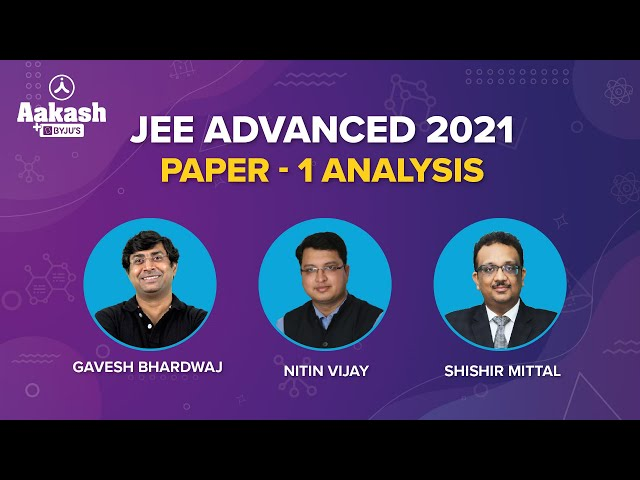s